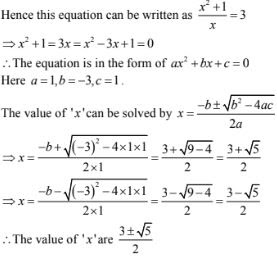# RD Sharma Solutions Chapter 8 Quadratic Equations Exercise 8.5 Class 10 Maths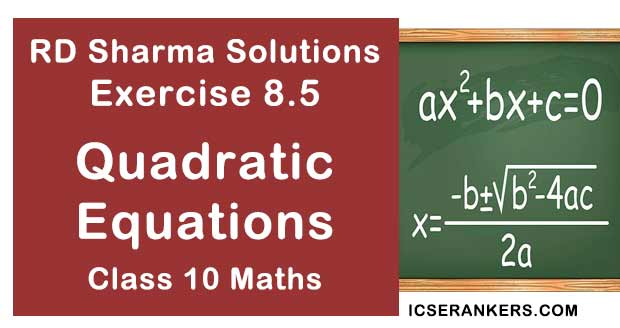Chapter Name RD Sharma Chapter 8 Quadratic Equations Book Name RD Sharma Mathematics for Class 10 Other Exercises Exercise 8.1Exercise 8.2Exercise 8.3Exercise 8.4Exercise 8.6 Related Study NCERT Solutions for Class 10 Maths

### Exercise 8.5 Solutions

1. Write the discriminant of the following quadratic equation:

(i) 2x2 - 5x + 3 = 0
(ii) x2 + 2x  + 4 = 0
(iii) (x - 1)(2x - 1) = 0
(iv) x2 - 2x + k = 0, K ∊ R
(v) √3x2 + 2√2x - 2√3 = 0
(vi) x2 - x + 1 = 0
(vii) 3x2 + 2x + k = 0
(viii) 4x2 - 3kx + 1 = 0

Solution

(i) 2x2 - 5x + 3 = 0
The given equation is in the form of ax2 + bx + c = 0
here a = 2, b = -5 and c = 3
The discriminant  D = b2 - 4ac
⇒ (-5)2 - 4×2×3
⇒ 25 - 24 = 1
∴ The discriminant of the following quadratic equation is 1.

(ii) x2 + 2x  + 4 = 0
The given equation is in the form of ax2 + bx +c = 0
here a= 1, b = 2 and c = 4
The discriminant is D = b2 - 4ac
⇒ (2)2 - 4 ×1 ×4
⇒ 4 - 16 = -12
∴ The discriminant of the following quadratic equation is -12.

(iii) (x - 1)(2x - 1) = 0
The given equation is (x - 1)(2x - 1) = 0
By solving it, we get 2x2 - 3x + 1 = 0
∴ This equation is in the form of ax2 + bx + c = 0
here a= 2, b = -3, c = -1
The discriminant is D = b2 - 4ac
⇒ (-3)2 - 4 × 2 ×1
⇒ 9 - 8 = 1
∴ The discriminant D, for the following quadratic equation is  1.

(iv) x2 - 2x + k = 0, K ∊ R
The given equation is in the form of  ax2 + bx + c = 0
Here a = 1, b = - 2, c = k [given k ∈ R]
The discriminant is D = b2 - 4ac
⇒ (-2)2 - 4×1 ×k
⇒ 4 - 4k
∴ The discriminant D, of the following quadratic equation is 4 - 4k, where K ∈ R

(v) √3x2 + 2√2x - 2√3 = 0
The given equation is in the form of ax2 + bx + c = 0
here a = √3, b = 2√2x and c = -2√3
The discriminant is D = (b2 - 4ac)
⇒ (2√2)2 - 4 × √3 × -2√3
⇒ 8 + 24 = 32
∴ The discriminant D, of the following quadratic equation is 32.

(vi) x2 - x + 1 = 0
The given equation is in the form of ax2 + bx + c = 0
here a = 1, b = - 1 and c = 1
The discriminant is D = b2 - 4ac
⇒ (-1)2 - 4×1×1
⇒1 - 4 = - 3
∴ The discriminant D, of the following quadratic equation is -3.

(vii) 3x2 +  2x + k = 0
The given equation has 3x2 + 2x + k = 0
here a = 3, b = 2, c = k
Given that the quadratic equation has real roots.
i.e., D = b2 - 4ac ≥ 0
⇒ 4 - 4 ×3 ×k ≥ 0
⇒ 4 - 12k ≥ 0
⇒ 4 ≥ 12k
⇒ 4 ≤ 4/12
⇒ k ≤ 1/3
The 'k' value should not exceed 1/3 to have the real roots for the given equation.

(viii) 4x2 - 3kx + 1 = 0
The given equation has 4x2 - 3kx + 1 = 0
here a = 3, b = 2, c = k
Given that quadratic equation has real roots
i.e., D = b2 - 4ac ≥ 0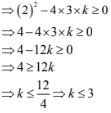2. In the following, determine whether the given quadratic equation have real roots and if so, find the roots:
(i) 16x2 = 24x + 1
(ii) x2 + x + 2 = 0
(iii) √3x2 + 10x - 8√3 = 0
(iv) 3x2 - 2x + 2 = 0
(v) 2x2 - 2√6x + 3 = 0
(vi) 3a2 x2 + 8abx + 4b2 = 0 , a ≠ 0
(vii) 3x2 + 2√bx - b = 0
(viii) x2  - 2x + 1 = 0
(ix) 2x2 + 5√3 + 6 = 0
(x) √2x2 + 7x + 5√2 = 0
(xi) 2x2 - 2√2x + 1 = 0
(xii) 3x2 - bx + 2 = 0

Solution

(i) 16x2 = 24x + 1
The given equation is in the form of 16x2 - 24x + 1 = 0
Hence, the equation is in the form of ax2 + bx + c = 0
Here, a = 16, b = -24, c = -1, D = b2 - 4ac = (-24)2 - 4 ×16 ×-1 = 576 + 64 = 640 > 0
As D > 0, the given equation has real roots, given by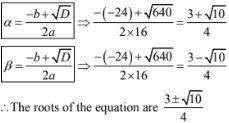(ii) x2 + x + 2 = 0
The given equation is in the form of ax2 + bx + c = 0
a = 1, b = 1, c = 2.
D = b2 - 4ac = (1)2 - 4×1×2 = 1 - 8 = -7 < 0
As Q < 0, the equation has no real roots

(iii) √3x2 + 10x - 8√3 = 0
The given equation is in the form of ax2 + bx + c = 0
here a = √3, b = 10 and c = -8√3(iv) 3x2 - 2x + 2 = 0
The given equation is in the form of ax2 + bx + c = 0
here a = 3, b = - 2,c = 2
The discriminant Q = b2 - 4ac
⇒ (-2)2  - 4×3×2 = 4 - 24
⇒ - 20 < 0
Hence as Q < 0.
The given equation has no real roots.

(v) 2x2 - 2√6x + 3 = 0
The given equation is in the form of ax2 + bx + c = 0
here a = 2, b = -2√6, c = 3
The discriminant Q = b2 - 4ac
⇒ (-2√6)2 - 4×2×3 = 24 - 24
⇒ 0
As Q = 0, the given equation has real and equal roots, They are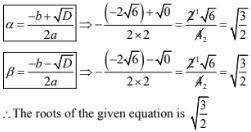(vi) 3a2 x2 +8abx + 4b2 = 0, a ≠ 0
The given equation is in the form of ax2 + bx +c = 0
here a = 3a2 ,b = 8ab, c = 4b2   [given a ≠ 0]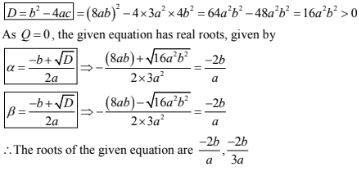(vii) 3x2 + 2√bx - b = 0
The given equation is in  the form of ax2 + bx + c = 0
here a = 3, b = 2√5, c = -5
The discriminant Q = b2 - 4ac
⇒ (2√5)2  -4 ×3 × -5 = 20 + 4 ×3 ×5
⇒ 20 + 60 - 80 > 0
As Q = 0, the given equation has real roots, given by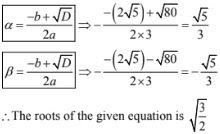(viii) x2 - 2x + 1 = 0
The given equation is in the form of ax2 + bx + c = 0
Here a = 1, b  = -2 and c = 1
The discriminant D = b2 - 4ac
⇒ (-2)2 - 4 ×1 ×1 = 0
As Q = 0, the given equation has real and equal roots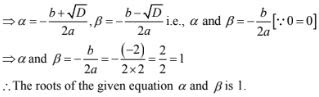(ix) 2x2 + 5√3 + 6 = 0
The given equation is in the form of ax2 + bx + c = 0
here a = 2, b = 5√3, c = 6
The discriminant D = b2 - 4ac
⇒ (5√3)2 - 4 ×2 ×6 = 75 - 48
⇒ 27 > 0
As Q = 0 , the given equation has real roots, given by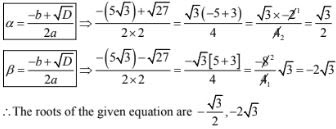(x) √2x2 + 7x + 5√2 = 0
The given equation is in the form of ax2 + bx + c = 0
here a = √2, b = 7, c = 5√2
The discriminant Q = b2 - 4ac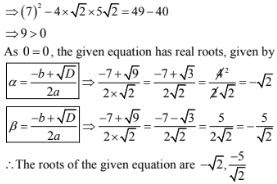(xi) 2x2 - 2√2x + 1 = 0
The given equation is in the form of ax2 + bx + c = 0
here a = 2, b = -2√2, c = 1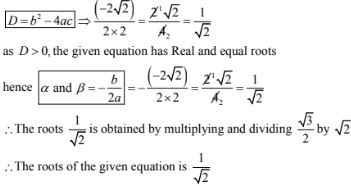(xii) 3x2 - bx + 2 = 0
The given equation is in the form of ax2 + bx + c = 0
here a = 3, b = -5, c = 2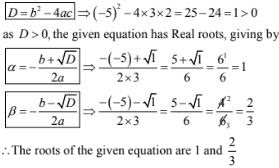3. Solve for x : (x - 1)/(x - 2) + (x - 3)/(x - 4) = 3(1/3); x ≠ 2, 4
Solution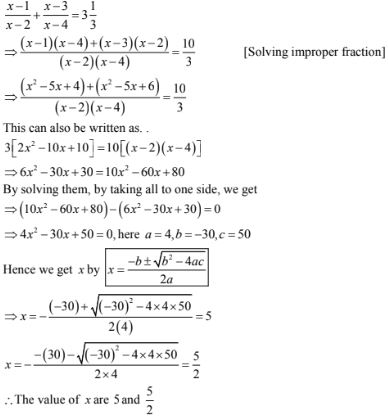4. Solve for x : 1/x - 1/(x- 7) = 3, x ≠ 0, 2
Solution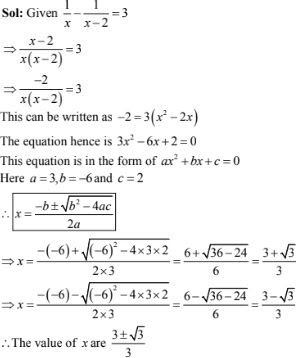5. x +1/x  = 3, x ≠ 0
Solution
Given x + 1/x = 3, x ≠ 0